Next: Electric Dipole Transitions Up: Time-Dependent Perturbation Theory Previous: Absorption and Stimulated Emission

# Spontaneous Emission of Radiation

So far, we have calculated the transition rates between two atomic states induced by external electromagnetic radiation. This process is known as absorption when the energy of the final state exceeds that of the initial state, and stimulated emission when the energy of the final state is less than that of the initial state. Now, in the absence of any external radiation, we would not expect an atom in a given state to spontaneously jump into a state with a higher energy. On the other hand, it should be possible for such an atom to spontaneously jump into an state with a lower energy via the emission of a photon whose energy is equal to the difference between the energies of the initial and final states. This process is known as spontaneous emission.

We can derive the rate of spontaneous emission between two atomic states from a knowledge of the corresponding absorption and stimulated emission rates using a famous thermodynamic argument initially formulated by Einstein . Consider a very large ensemble of similar atoms placed inside a closed cavity whose walls (which are assumed to be perfect emitters and absorbers of radiation) are held at the constant temperature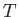. Let the system have attained thermal equilibrium. According to statistical thermodynamics, the cavity is filled with so-called black-body electromagnetic radiation whose energy spectrum is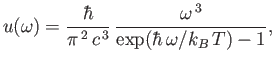(8.153)

where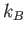is the Boltzmann constant. This well-known result was first obtained by Max Planck in 1900 [82,83].

Consider two atomic states, labeled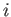and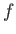, with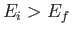. One of the tenants of statistical thermodynamics is that in thermal equilibrium we have so-called detailed balance. (See Section 8.8.) This means that, irrespective of any other atomic states, the rate at which atoms in the ensemble leave statedue to transitions to stateis exactly balanced by the rate at which atoms enter statedue to transitions from state. The former rate (i.e., number of transitions per unit time in the ensemble) is written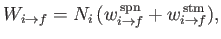(8.154)

where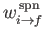and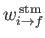are the rates of spontaneous and stimulated emission, respectively, (for a single atom) between statesand, and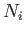is the number of atoms in the ensemble in state. Likewise, the latter rate takes the form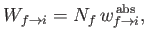(8.155)

where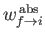is the rate of absorption (for a single atom) between statesand, and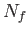is the number of atoms in the ensemble in state. The previous expressions describe how atoms in the ensemble make transitions from stateto statevia a combination of spontaneous and stimulated emission, and make the reverse transition as a consequence of absorption. In thermal equilibrium, we have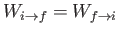, which gives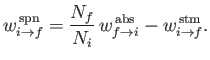(8.156)

Equations (8.149) and (8.150) imply that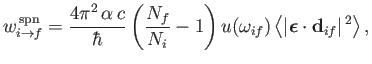(8.157)

where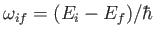, and the large angle brackets denote an average over all possible polarization directions of the incident radiation (because, in equilibrium, the radiation inside the cavity is isotropic). In fact, it is easily demonstrated that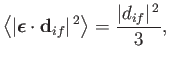(8.158)

where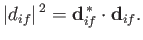(8.159)

(See Exercise 7.) Now, another famous result in statistical thermodynamics is that, in thermal equilibrium, the number of atoms in an ensemble occupying a state of energy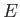is proportional to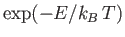. This implies that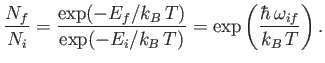(8.160)

Thus, it follows from Equations (8.156), (8.160), (8.161), and (8.163) that the rate of spontaneous emission between statesandtakes the form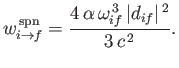(8.161)

Note, that, although the previous result has been derived for an atom in a radiation-filled cavity, it remains correct even in the absence of radiation.

The direction of propagation of a photon spontaneously emitted by an atom is specified by its normalized wavevector,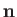. Likewise, the electric polarization direction of the photon is specified by the unit vector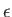. However,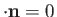, which implies that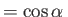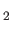, where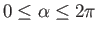. Here, the unit vectors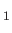,, andare mutually perpendicular, and form a right-handed set (i.e.,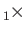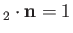). The vectorsandrepresent the two possible independent electric polarization directions of the emitted photon. Equation (8.164) generalizes to give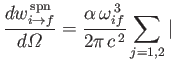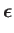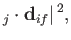(8.162)

where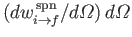is the rate of spontaneous emission of photons whose propagation directions lie in the range of solid angle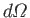. Of course,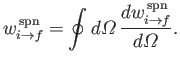(8.163)

(See Exercise 8.)Next: Electric Dipole Transitions Up: Time-Dependent Perturbation Theory Previous: Absorption and Stimulated Emission
Richard Fitzpatrick 2016-01-22